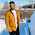Java Program for Moving Ball with Applet

Tip Abstraction:
This Java program code shows an output of animated moving ball with live output

This is a simple and funny java applet program for an animated free hand moving ball. Actually it is not a ball; it is rounded oval, filled with color black. This ball will bounce continuously without any pause or stop. You have to close your applet viewer or browser to stop the movement of this unstoppable moving ball. That is, here infinity loops are used.

Infolet made this program using applet, you can do this program in ‘Frame’ by extending frame. Then you have to write code for main for this program.
(Learn here how to make and run a java applet program).

Program code:
 import java.applet.*; import java.awt.*;  /* */ public class ball extends Applet implements Runnable  {  Thread t;  int x = 0;  int y = 0; public void start()  {  t = new Thread(this);  t.start();  } public void paint(Graphics g)    {       g.fillOval(x,y,100,100);     } public void run()  {    try       {     for(;;)       {              for(;;)            {                if(y == 120)                    {                     break;                    }  else if (x == 390)      {  x = 0;  y = 0; repaint(); }                    else                    {                     y = y +3;                     x = x +3;                      Thread.sleep(100);             repaint();              }               }         for(;;)              {              if(y==0)                  {                         break;                         }               else if (x == 390)        {       x = 0; y = 0; repaint(); } else                 {  y = y-3;             x = x +3;             Thread.sleep(100);               repaint();              }                }            run();        }   } catch(InterruptedException e)     {     }  } }

Live Output:
If your browser contains java and compatible with JRE, then you can watch live moving ball output of above program code below.

Or, Screenshot of output:

Ø If you like this program, please like us here..

1.Pls, Explain me
for(;;)
{
------
------
}
What is meaning of above code?

1.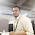it's a infinite loop

2.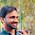for(;;) means infinity loop; never end

3.what if i want 2 such balls to be moving in same applet

4.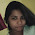thnks fr sharing vry helpful 2 me

5.Very good job..
But if we give 50 delay then ball do not blink

6.Very good
But if we give 50ms time then ball look clear..

7.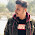Here is my code.....

import java.applet.*;
import java.awt.*;
public class Ball extends Applet implements Runnable
{
int x=0;
int y=0;
public void start()
{
t.start();
}
public void paint(Graphics g)
{
g.fillOval(x,y,100,100);
}
public void run()
{
try
{
for(;;);
{
for(;;);
{
if(y==120)
{
break;
}
else if(x==390)
{
x=0;
y=0;
repaint();
}
else
{
x=x+3;
y=y+3;
repaint();
}
}
for(;;)
{
if (y==0)
{
break;
}
else if(x==390)
{
x=0;
y=0;
repaint();
}else
{
y=y-3;
x=x+3;
repaint();
}
}
run();
}
}
catch(Exception e)
{}
}
}

and the error shows in 27th line is:
Ball.java:27: error: break outside switch or loop
break;
^
1 error
1.